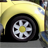# Extract By Mask emptied my attribute table

2134
0
04-18-2014 07:30 AMNew Contributor III
I've been trying to automate the process of clipping thematic rasters to the same extent and then subdividing them by polygon. However, there have been a lot of errors along the way. Here's the latest:

This occurs at the beginning of the 2nd iteration of the for loop. Examination of the data layers shows that the attribute tables of masked_themes are being emptied by the 1st iteration, for an unknown reason. (The headers are still there.)

```import matplotlib.pyplot as plt
arcpy.env.workspace = 'in_memory'
arcpy.env.snapRaster = r'Fr Demo\Q70slope'
arcpy.env.cellSize = r'Fr Demo\Q70slope'
# store working lists
provinces = [r'Physiographic Provinces\PhysioPacific',r'Physiographic Provinces\PhysioAtlantic',r'Physiographic Provinces\PhysioCentral',r'Physiographic Provinces\PhysioDarien']
themes = ['HWSDunit1kmWGS','Geo1kmWGS',r'Fr Demo\Q70slope']
validators = [r'USGS\Combined\MitchCatBinary1kmGCS',r'U of Oslo\NicCatBinary1kmGCS',r'El Salvador\1km rasters\EScat1kmGCSBinaryClip',r'GLC\GLC1kmGCSbinary']
trainers = [r'Training\TrainingWeighted1kmGCS',r'Training\TrainingAll1kmGCS',r'Training\TrainingSampled1kmGCS']

# create presence raster
temp = arcpy.sa.IsNull(raster)+1
temp2 = arcpy.sa.LessThan(temp,2)
del temp, temp2

for raster in rasters:
del temp

for i in range(len(rasters)):
if i == 0: mask = arcpy.sa.Times(rasters,1)

outputs = []
for raster in rasters:
outputs.append(temp)
del temp
return outputs

def clip_to_shape(rasters,shape):
outputs = []
for raster in rasters:
outputs.append(temp)
del temp
return outputs

def get_Fr_table(theme,landslides):
fieldname = "value"
newfieldname = 'Fr'
zsum_table = arcpy.sa.ZonalStatisticsAsTable(theme, fieldname, landslides, 'temp', 'DATA', 'SUM')
rows = arcpy.UpdateCursor(zsum_table)
total_count, total_sum = 0,0
for row in rows:
total_count += row.getValue("count")
total_sum += row.getValue("sum")
rows = arcpy.UpdateCursor(zsum_table)
for row in rows:
Lcb = row.getValue("sum")
Scb = row.getValue("count")
Fr = (float(Lcb)/total_sum)/(float(Scb)/total_count)
row.setValue(newfieldname, Fr)
rows.updateRow(row)
return zsum_table

# create raster mask for all layers